Time: 3 Hours                                                                                                     Max. Marks: 100

NOTE: There are 9 Questions in all.

·      Question 1 is compulsory and carries 20 marks. Answer to Q.1 A. must be written in the space provided for it in the answer book supplied and nowhere else.

·      Out of the remaining EIGHT Questions answer any FIVE Questions. Each question carries 16 marks.

·      Any required data not explicitly given, may be suitably assumed and stated.

Q.1 A.   Choose the correct or best alternative in the following:                                         (2x10)

a.       n bits in operation code imply that there are ___________ possible distinct operators

(A)  2n                                                (B)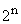(C)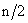(D)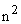b.      ___________ register keeps track of the instructions stored in program stored in memory.

(A)    AR (Address Register)                 (B)  XR (Index Register)

(C)  PC (Program Counter)                 (D)  AC (Accumulator)

c.   Memory unit accessed by content is called

(A)     Read only memory                      (B)  programmable memory

(C)   virtual memory                             (D)  associative memory

d.   ‘Aging registers’ are

(A)   counters which indicate how long ago their associated pages have been

referenced.

(B)   registers which keep track of when the program was last accessed.

(C) counters to keep track of last accessed instruction.

(D) counters to keep track of the latest data structures referred.

e.   The instruction ‘ORG O’ is a

(A)     machine instruction.                      (B)  pseudo instruction.

(C)  high level instruction.                     (D)  memory instruction.

f.    Translation from symbolic program into Binary is done in

(A)     Two passes                                  (B)  Directly

(C)  Three passes                                (D)  Four passes

g.   A floating point number that has a 0 in the MSB of mantissa is said to have

(A)     overflow                                      (B)  underflow

(C)  improper number                          (D)  undefined

h.   The BSA instruction is

(A)    Branch and store accumulator

(B)    Branch and save return address

(D)    Branch and show accumulator

i.    Which of the following is a main memory

(B)     Secondary memory.                     (B)  Auxiliary memory.

(C)  Cache memory.                            (D)  Virtual memory.

j.    The 2’s compliment form (Use 6 bit word) of the number 1010 is

(A)  111100.                                       (B)  110110.

(C)  110111.                                       (D)  1011.

Answer any FIVE Questions out of EIGHT Questions.

Each question carries 16 marks.

Q.2     a.   What binary number does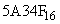represent?                                                  (1)

b.   Using NAND gate generate the NOT, AND, OR and NOR functions.      (2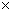4=8)

c.   Minimize the expression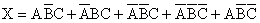Using Karnaugh’s map.                                                                                      (4)

d.   Subtract 1010100 – 1000011 using 2’s complement.                                         (3)

Q.3     a.   For the following memory units (specified by the number of words the number of bits per word), determine the number of address lines, Input / Output lines and the number of bytes that can be stored in the specified memory

(i)                  64K8

(ii)                16M32

(iii)               4G64

(iv)              2K16                                                                    (1½4 = 6)

b.   What is a micro-operation?  List and briefly explain the most commonly encountered arithmetic operations.                                                            (1+4)

c.   Discuss the different ways in which ROM can be programmed.                           (5)

Q.4     a.   Design a 4 bit arithmetic circuit which is capable of performing the following micro-operations:-

Subtraction (with and without borrow)

Increment

Decrement

and Transfer

Substantiate the circuit diagram with the help of an example.                              (12)

b.   Give the steps required to insert a new value into a group of bits.  Using the above method change the decimal number 106 to 154.                (2+2=4)

Q.5     a.   A computer uses a memory unit with 256 K words of 32 bits each.  A binary instruction code is stored in one word of memory.  The instruction has four parts: an indirect bit, an operation code, a register code part to specify one of 64 registers and an address part

(i)                  How many bits are there in operation code, the register code part and the address part.

(ii)                Draw the instruction word format and indicate the number of bits in each part.                    (2+2)

b.   Draw a detailed flowchart of the instruction cycle.  Indicate the conditions in which register-reference / memory-reference and input-output instructions are executed.  Also include the interrupt cycle micro-operations in the flowchart.                                                                               (9)

c.   How are the effective addresses computed when the instructions are

(i)   Immediate.                                    (ii)  Direct.

(iii) Indirect.                                                                                               (1+1+1)

Q.6     a.   What do you understand by term “addressing mode”?  Explain why do we need different addressing modes.  Name two addressing modes that need no address field at all.                                            (1+2+1)

b.   Discuss the major reasons that can cause the instruction pipeline to derivate from its normal operation.                                                                   (4)

c.   Given the cache access time as 10 ns memory access time as 100 ns and cache hit rate as 90%, calculate the effective memory access time.              (3)

d.   Explain the terms burst transfer and cycle stealing.                                               (5)

Q.7     a.   Explain how DMA controller communicates and transfers data between the peripheral devices and RAM.                                                                (8)

b.   Differentiate between

(i)                  Isolated and Memory-Mapped I/O.

(ii)                Associative Mapping and Direct Mapping.                                             (8)

Q.8     a.   Draw the flowchart to add two numbers in signed magnitude form.                      (5)

b.   What is a page fault?  What does a page fault signify?  Explain the different page replacement algorithms which determine the page to be removed in case of full memory.                                         (6)

c.   What is the disadvantage of transferring data through strobe control method?  Which method overcomes this disadvantage?  Explain.                        (2+1+2)

Q.9     a.   What is the significance of initialising cache?  How is it done?                              (4)

b.   How is source Initiated Handshaking different from Destination Initiated Handshaking?  Explain the two through Block & Timing diagrams.       (8)

c.   Why do peripherals need special communication links to interface them with central processing unit?                                                                      (4)